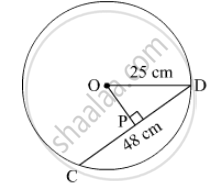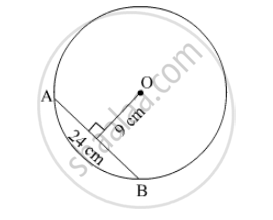# SSC (English Medium) 8th Standard - Maharashtra State Board Question Bank Solutions for Mathematics

Subjects
Topics
Subjects
Popular subjects
Topics
Mathematics
< prev  41 to 60 of 360  next >

Expand.

(m+2/3)(m-7/3)

[0.05] Expansion Formulae
Chapter: [0.05] Expansion Formulae
Concept: Expansion of (x + a)(x + b)

Expand.

(x+1/x)(x-1/x)

[0.05] Expansion Formulae
Chapter: [0.05] Expansion Formulae
Concept: Expansion of (x + a)(x + b)

Expand.

(1/y+4)(1/y-9)

[0.05] Expansion Formulae
Chapter: [0.05] Expansion Formulae
Concept: Expansion of (x + a)(x + b)

Radius of a circle with centre O is 25 cm. Find the distance of a chord from the centre if length of the chord is 48 cm.[0.17] Circle - Chord and Arc
Chapter: [0.17] Circle - Chord and Arc
Concept: Properties of Chord of a Circle

O is centre of the circle. Find the length of radius, if the chord of length 24 cm is at a distance of 9 cm from the centre of the circle.[0.17] Circle - Chord and Arc
Chapter: [0.17] Circle - Chord and Arc
Concept: Properties of Chord of a Circle

C is the centre of the circle whose radius is 10 cm. Find the distance of the chord from the centre if the length of the chord is 12 cm.

[0.17] Circle - Chord and Arc
Chapter: [0.17] Circle - Chord and Arc
Concept: Properties of Chord of a Circle

Find the volume of a box if its length, breadth and height are 20 cm, 10.5 cm and 8 cm respectively.

[0.16] Surface Area and Volume
Chapter: [0.16] Surface Area and Volume
Concept: Standard Unit of Volume

A cuboid shape soap bar has volume 150 cc. Find its thickness if its length is 10 cm and breadth is 5 cm.

[0.16] Surface Area and Volume
Chapter: [0.16] Surface Area and Volume
Concept: Standard Unit of Volume

How many bricks of length 25 cm, breadth 15 cm and height 10 cm are required to build a wall of length 6 m, height 2.5 m and breadth 0.5 m?

[0.16] Surface Area and Volume
Chapter: [0.16] Surface Area and Volume
Concept: Standard Unit of Volume

For rain water harvesting a tank of length 10 m, breadth 6 m and depth 3 m is built. What is the capacity of the tank? How many litre of water can it hold?

[0.16] Surface Area and Volume
Chapter: [0.16] Surface Area and Volume
Concept: Standard Unit of Volume

Lengths of the diagonals of a rhombus are 15 cm and 24 cm, find its area.

[0.15] Area
Chapter: [0.15] Area
Concept: Area of a Rhombus

Lengths of the diagonals of a rhombus are 16.5 cm and 14.2 cm, find its area.

[0.15] Area
Chapter: [0.15] Area
Concept: Area of a Rhombus

If perimeter of a rhombus is 100 cm and length of one diagonal is 48 cm, what is the area of the quadrilateral ?

[0.15] Area
Chapter: [0.15] Area
Concept: Area of a Rhombus

If length of a diagonal of a rhombus is 30 cm and its area is 240 sq cm, find its perimeter.

[0.15] Area
Chapter: [0.15] Area
Concept: Area of a Rhombus

Factorise.

x+ 9x + 18

[0.06] Factorisation of Algebraic Expressions
Chapter: [0.06] Factorisation of Algebraic Expressions
Concept: Factorisation of a Quadratic Trinomial by Splitting the Middle Term

Factorise.

x− 10x + 9

[0.06] Factorisation of Algebraic Expressions
Chapter: [0.06] Factorisation of Algebraic Expressions
Concept: Factorisation of a Quadratic Trinomial by Splitting the Middle Term

Factorise.

y+ 24y + 144

[0.06] Factorisation of Algebraic Expressions
Chapter: [0.06] Factorisation of Algebraic Expressions
Concept: Factorisation of a Quadratic Trinomial by Splitting the Middle Term

Factorise.

5y+ 5y − 10

[0.06] Factorisation of Algebraic Expressions
Chapter: [0.06] Factorisation of Algebraic Expressions
Concept: Factorisation of a Quadratic Trinomial by Splitting the Middle Term

Factorise.

p− 2p − 35

[0.06] Factorisation of Algebraic Expressions
Chapter: [0.06] Factorisation of Algebraic Expressions
Concept: Factorisation of a Quadratic Trinomial by Splitting the Middle Term

Factorise.

p− 7p − 44

[0.06] Factorisation of Algebraic Expressions
Chapter: [0.06] Factorisation of Algebraic Expressions
Concept: Factorisation of a Quadratic Trinomial by Splitting the Middle Term
< prev  41 to 60 of 360  next >